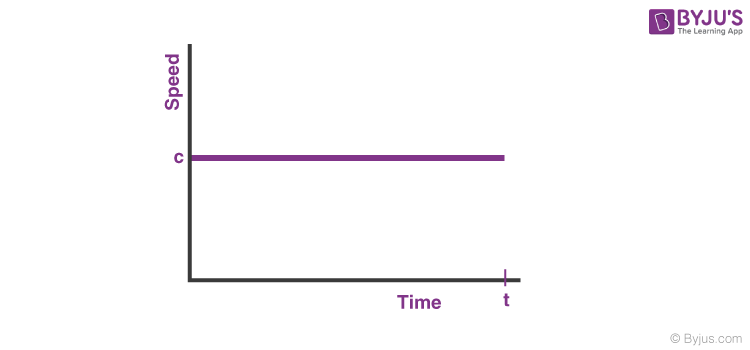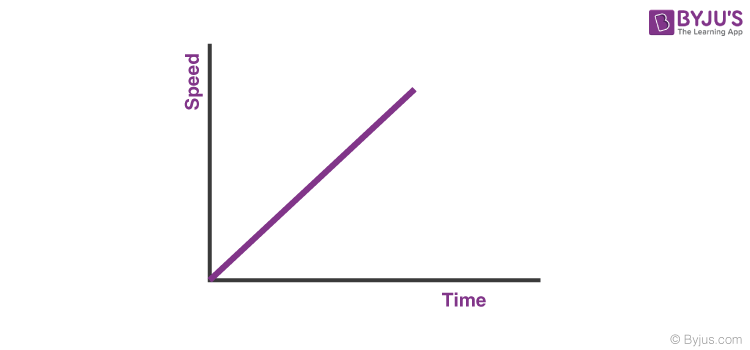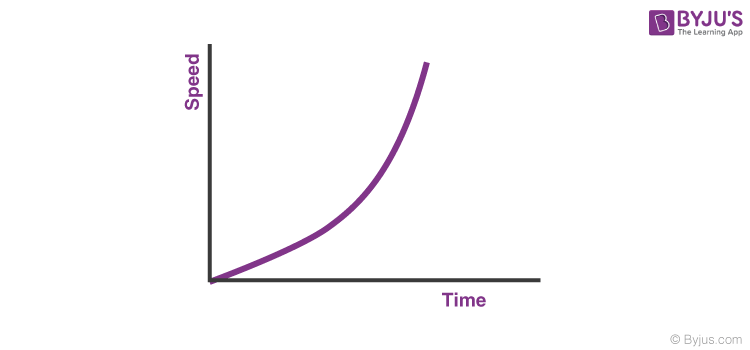# The area under the Speed-Time graph gives the _______

The area under the speed-time graph gives the distance travelled.

## Speed-Time Graph

• When explaining the movement of an object, speed-time graphs are extremely useful.
• They can be used to decide whether an object is moving or not at any given time.
• They can also be used to determine the speed at which an object is moving at any given time.
• We can measure any acceleration, change in speed, and change in time using data from the graph.
• We can also use graphs to figure out how far we’ve travelled. The area under a speed-time graph represents the distance travelled.

## Speed-time graphs with constant speed## Speed-time graphs with constant acceleration### Speed-time graphs with increasing acceleration(13)(1)